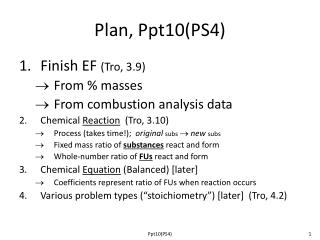DownloadDownload PresentationPlan, Ppt10(PS4)

# Plan, Ppt10(PS4)

Download Presentation## Plan, Ppt10(PS4)

- - - - - - - - - - - - - - - - - - - - - - - - - - - E N D - - - - - - - - - - - - - - - - - - - - - - - - - - -
##### Presentation Transcript

1. Plan, Ppt10(PS4) • Finish EF (Tro, 3.9) • From % masses • From combustion analysis data • Chemical Reaction (Tro, 3.10) • Process (takes time!); originalsubs  newsubs • Fixed mass ratio of substances react and form • Whole-number ratio of FUs react and form • Chemical Equation (Balanced) [later] • Coefficients represent ratio of FUs when reaction occurs • Various problem types (“stoichiometry”) [later] (Tro, 4.2) Ppt10(PS4)

2. Empirical Formula (EF) Quick Review • Need to find the subscripts => lowest whole-number ratio of atoms => lowest whole-number ratio of MOLES (of atoms) Ppt10(PS4)

3. Determining the Empirical Formula of a Compound •  NOTE: If molar mass is also given, it may be easiest to use that mass (rather than 100 g). You will then get the molecular formula first (which can then be reduced, if needed) • From mass data (of each element A, B, C, …) of a sample: masses (of A, B, C, …)  moles (of A, B, C, … atoms)  ratio of molesreduced(whole-number) ratio of moles  formula (subscripts) • From % mass data (of each element A, B, C, …) • Get mass data, and do as above! • Assume 100 g of compound for convenience • 20.4 % of 100 g = 20.4 g! (etc.) Ppt10(PS4)

4. From Green Handout Sheet (PS4 & 5 Practice) • What is the empirical formula of tetraethyllead, the “lead” in gasoline? The composition is: 29.71% C, 64.06% Pb, 6.23% H (These percentages are by mass) Ppt10(PS4)

5. PS Sign-Posting The concepts and skills related toproblems14, 18, and 19 on PS4have been covered now in this PowerPoint. Give those problems a try now! Ppt10(PS4)

6. From Combustion Analysis Data • Often cannot easily get the elements out • So, react with oxygen: • All C atoms end up in CO2 molecules! • All H atoms end up in H2O molecules! • Figure out C in sample from mass of CO2 • Figure out H in sample from mass of H2O • Figure out a 3rd atom type (O, N, S, …) by mass difference (as in Exp 4—get Cl “by difference”) Ppt10(PS4)

7. Combustion Analysis Figure 3.10 e.g., excess O2, sulfur oxides, nitrogen oxides, halogen-oxides , etc. e.g., Mg(ClO4)2 e.g., NaOH Ppt10(PS4)

8. A hydrocarbon of unknown formula CxHy was submitted to combustion analysis with the following results. What is the empirical formula of the hydrocarbon? From Green Handout Sheet (PS4 & 5 Practice) Ppt10(PS4)

9. From Green Handout Sheet (PS4 & 5 Practice) • Coniine, a toxic substance isolated form poison hemlock, contains only carbon, hydrogen, and nitrogen. Combustion analysis of a 5.024 g sample yields 13.90 g of CO2 and 6.048 g of H2O. What is the empirical formula of coniine? Ppt10(PS4)

10. PS Sign-Posting The concepts and skills related toproblem 17 on PS4have been covered now in this PowerPoint. Give those problems a try now! [end of PS4 material] Ppt10(PS4)

11. Discussion of Meaning of a Balanced Chemical Equation • Chemical Reaction • Initial substances (reactants) turn into new substances (products) • Takes time (a process) • Involves actual amounts • Can be expressed in a variety of ways: grams, moles, L, etc. • Chemical Equation • Represents(a type of) chemical reaction (but not “literally”) • Symbols (not “reality”), on paper • Formulas, coefficients, an arrow • Does not involve actual amounts! Only RATIOof combination and formation • Reaction vs. equation (different!) • Most chemistry texts tend to discuss this initially but then use the words interchangeably later on(!) Be careful!  Ppt10(PS4)

12. Discussion of Meaning of a Balanced Chemical Equation (Example) Chemical Reaction 4 g of methane react with 16 grams of oxygen to produce 11 grams of carbon dioxide and 9 grams of water, all as gases. Chemical Equation CH4(g) + 2O2(g)  CO2(g)+2H2O(g) • Coefficients represent a ratio only, and they represent the ratio of formula units, most fundamentally. • Here, a 1 : 2 : 1 : 2 ratio of molecules of CH4, O2, CO2, and H2O but onlyas reaction occurs. The reaction occurs over some period of time, during which the number of molecules of methane and oxygen steadily decrease as the number of molecules of carbon dioxide and water increase. Ppt10(PS4)

13. Discussion of Meaning of a Balanced Chemical Equation • Analogy (hypothetical): Working at Lawson products 25 boxes packed up → \$15 in pocket • How much money is in your pocket after the “reaction” occurs? • The equationalone can’t answer that! • How much “reaction” occurred? (not indicated by equation!) • How much was in your pocket before the reaction? Ppt10(PS4)